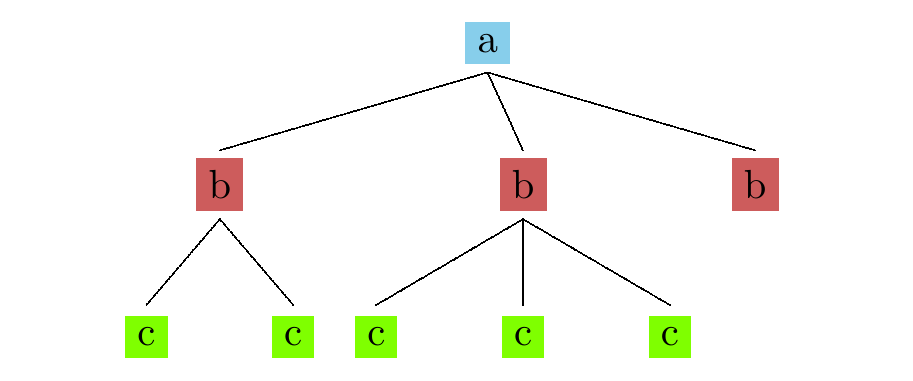## Nondeterminism

Tikhon Jelvis (tikhon@jelv.is)

Zoom in/out: Ctrl or Command + +/-

# 1 Function Composition

• function composition animation
• `a → b`, `b → c`, `a → c`
• one function after another
• directly pass results in between
• `x ⇒ a`
• `f ⇒ b`
• `g ⇒ c`

• nondeterministic composition animation
• customize function composition
• “different shape” of function
• `a → m b` vs `a → b`
• `m` is a “slot” to insert custom behavior

# 3 Lists

• consider `m` as `[]`
• `a → [b]`, `b → [c]`, `a → [c]`
• different shape: tree!
• `a → [b]`
• nondeterministic function from `a` to `b`

# 4 List Composition

• `f <=< g`
```f      ⇒ [b]
x      ⇒ a
map g  ⇒ [[c]]
concat ⇒ [c]
```
• so for lists, `join = concat`

# 5 A Lazy Tree• result: `[c, c, c, c, c]`
• lazy

# 6 Map Coloring# 7 Map Coloring# 8 Step

```step :: Coloring -> Vertex -> [Coloring]
step graph vertex =
map (color vertex) possible
where possible =
colors \\ neighbors vertex coloring
```
• try every available color
• if none: returns `[]`
• branch terminated

# 9 foldM

```listToMaybe \$ foldM step emptyColoring nodes
```
• `foldM` encapsulates backtracking logic
• like nested loops
• `listToMaybe``safeHead`
• all works thanks to laziness